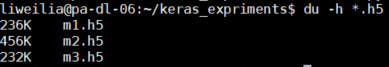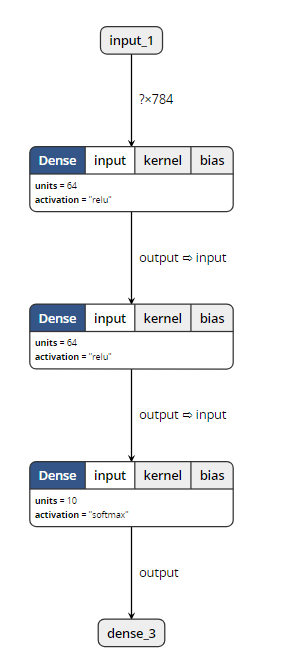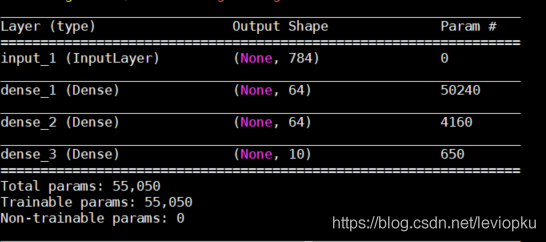# Keras saves save() and save in the model_ weights()

Today, we did an experiment on the model saved by keras, hoping to help you understand the differences between the models saved by keras.

We know that the model of keras is usually saved as a file with the suffix H5, such as final_ model.h5。 The same H5 file uses save () and save_ The effect of weight () is different.

We use MNIST, the most common data set in the universe, to do this experiment

``````inputs = Input(shape=(784, ))
x = Dense(64, activation='relu')(inputs)
x = Dense(64, activation='relu')(x)
y = Dense(10, activation='softmax')(x)

model = Model(inputs=inputs, outputs=y)``````

Then, import MNIST data for training, and save the model in two ways. Here, I also save the untrained model, as follows:

``````from keras.models import Model
from keras.layers import Input, Dense
from keras.datasets import mnist
from keras.utils import np_utils

(x_train, y_train), (x_test, y_test) = mnist.load_data()
x_train=x_train.reshape(x_train.shape,-1)/255.0
x_test=x_test.reshape(x_test.shape,-1)/255.0
y_train=np_utils.to_categorical(y_train,num_classes=10)
y_test=np_utils.to_categorical(y_test,num_classes=10)

inputs = Input(shape=(784, ))
x = Dense(64, activation='relu')(inputs)
x = Dense(64, activation='relu')(x)
y = Dense(10, activation='softmax')(x)

model = Model(inputs=inputs, outputs=y)

model.save('m1.h5')
model.summary()
model.compile(loss='categorical_crossentropy', optimizer='sgd', metrics=['accuracy'])
model.fit(x_train, y_train, batch_size=32, epochs=10)
#loss,accuracy=model.evaluate(x_test,y_test)

model.save('m2.h5')
model.save_weights('m3.h5')
``````

As you can see, I have saved m1.h5, m2.h5 and m3.h5 files. So, let’s see what’s the difference between these three things. First, look at the size:M2 represents the result of the model saved by save (), which not only keeps the graph structure of the model, but also saves the parameters of the model. So it’s the biggest.

M1 represents the result of the model before training saved by save (). It saves the graph structure of the model, but it should not save the initialization parameters of the model, so its size is much smaller than m2.

M3 means save_ Weights () saves the results of the model. It only saves the parameters of the model, but it does not save the graph structure of the model. So it’s much smaller than m2.

Through the visualization tool, we found that: (open M1 and M2 can show the following structure)When opening m3, the visualization tool reported an error. So it can be proved that save_ Weights () does not contain model structure information.

Loading model

The model files saved by two different methods also need different loading methods.

``````from keras.models import load_model

model = load_model('m1.h5')
#model = load_model('m2.h5')
#model = load_model('m3.h5')
model.summary()
``````

Only when loading m3. H5, this code will report an error. Other outputs are as follows:It can be seen that only the H5 file saved by save() can be downloaded directly_ Model () open!

So, how can we open the saved parameter (M3. H5)?

This is a little more complicated. Because m3 does not contain model structure information, we need to describe the model structure again before loading m3, as follows:

``````from keras.models import Model
from keras.layers import Input, Dense

inputs = Input(shape=(784, ))
x = Dense(64, activation='relu')(inputs)
x = Dense(64, activation='relu')(x)
y = Dense(10, activation='softmax')(x)

model = Model(inputs=inputs, outputs=y)
model.load_weights('m3.h5')``````

The above m3 into M1 and M2 is no problem! It can be seen that the model saved by save () has obvious advantages except that it takes up more memory. Therefore, in the case of no lack of hard disk space, it is recommended that you use save () to save more.

be careful! If you want to load_ Weights (), you must ensure that the calculation structure with parameters described by you is completely consistent with that in H5 file! What is parametric computing structure?Just fill in the parameter pit. We changed the above non parametric structure and found that H5 files can still be loaded successfully. For example, changing softmax to relu does not affect the loading.

For save() and save() of keras_ No problem at all